# Working of Backpropagation in Neural Networks and Deep Learning

While training an Artificial Neural Network, data samples are passed forward and backwards through the network multiple times. It’s the same as a cricketer getting trained to play cover drive. During this training, the same length ball is bowled to him, and he tries to master that stroke. Similarly, machines see the same data samples to understand the underlying patterns present in the data.

In our forward propagation in the ANN blog, we discussed how a data sample gets transformed into the desired output format through multiple rounds of transformations or matrix multiplications. In this blog, we will discuss one of the most famous and fundamental concepts, i.e., backward propagation.

#### Key takeaways from this blog

After going through this blog, we will be able to understand the following things:

1. What is backward propagation, and what is its importance?
2. The mathematics involved in backward propagation.
3. The role of an optimization algorithm in backward propagation.

#### What is backpropagation, and what is its significance in Neural Networks?

In Machine Learning, machines perform actions, analyze their mistakes, and then try to rectify them to achieve perfection. We provide an input sample to the machine and ask them to complete a forward pass. During this forward pass, the input sample gets transformed into the output. This output may be different from what we expected.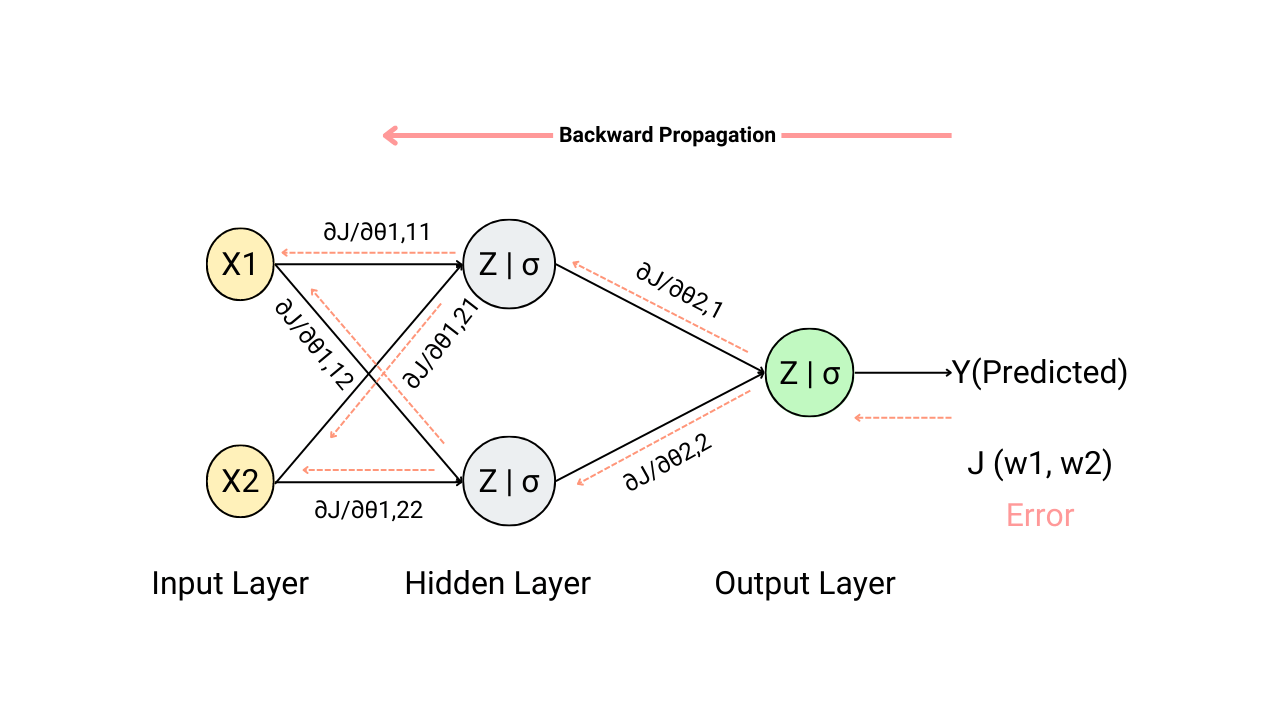As Neural Networks are supervised learning algorithm, it already knows the perfect output corresponding to any input provided. So, machines calculate the error between the ideal output and the output received from the forward pass. A forward pass can inform machines about their prediction mistakes on the given input sample. But there is no intelligence if machines don’t do anything to rectify those mistakes.

Hence, after performing the forward pass, machines send back the errors in the form of cost value and analyze them to perform rectifications. This rectification is nothing but updating the parameters involved in the forward pass to transform the input sample into output.

Here the cost values are sent “backwards” towards the input sample, and hence this propagation is known as “backward propagation”. The primary reason for sending back the cost values is to calculate the gradients that the optimization algorithms will use to learn parameters.

### Selecting the architecture for ANNs

Before moving towards the backpropagation step in Neural Networks, let’s quickly list theories and their references that will be used to perform backpropagation. To solve any problem statement via ANNs, we first need to design a meaningful architecture. If one wants to revise these concepts, references for the topics are also mentioned. Designing ANN involves:

#### Selecting the number of hidden layers required and the number of nodes in the hidden layer/s and the output layer.

In our components of neural network and introduction to neural network blogs, we have discussed ways to decide the number of nodes in the input layer, hidden layer/s and output layer. Selecting the number of hidden layers depends upon many factors: the complexity of the problem statement, the availability of compute power and whether the model is underfitting or overfitting.

The deeper (more hidden layers) the network will be, the more complexities will come during the backpropagation step.

#### Choosing the appropriate activation function for the hidden layer and the output layer.

Selecting an appropriate activation function is one of the most important steps in designing ANNs. We have discussed factors that influence the selection of activation functions for hidden layers and the selection of activation functions for the output layer. We represented the formula for the popular activation functions and their derivatives in all these articles. These derivatives will play a crucial role during the backward propagation (backpropagation) step, and we will see that in a while.

#### Forward Propagation of the data samples

While training, data samples pass through multiple rounds of forward and backward propagation. As we said, backpropagation is sending back the cost function backwards into the network, but to calculate the cost function, we first need to perform the forward propagation. We will be using the concepts of forward propagation in the later part of this blog, so if you want a detailed discussion on this, please read our blog on the introduction to forward propagation in neural networks.

#### Finding the objective function for the Neural Network.

Machines don’t understand the overall objective for which we are building an ANN model. For example, if we say machines in plain English to segregate spam and non-spam emails, it won’t be able to understand. We need to convey this problem to machines in a language that machines can understand. This translation of our machine learning problem statement into a form in which machines can understand (and solve) is the prime goal in any Machine Learning process.

We represent our overall problem statement through loss and cost functions. There is one famous quote in Neural Smithing Book: “It is important that our loss/cost function faithfully represent our design goals. If we choose a poor loss/cost function and obtain unsatisfactory results, the fault is ours for badly specifying the goal of the search.” One can visit our blog on poplar loss and cost functions for classification and regression problems to better understand how to translate our problem statement in a machine-readable format.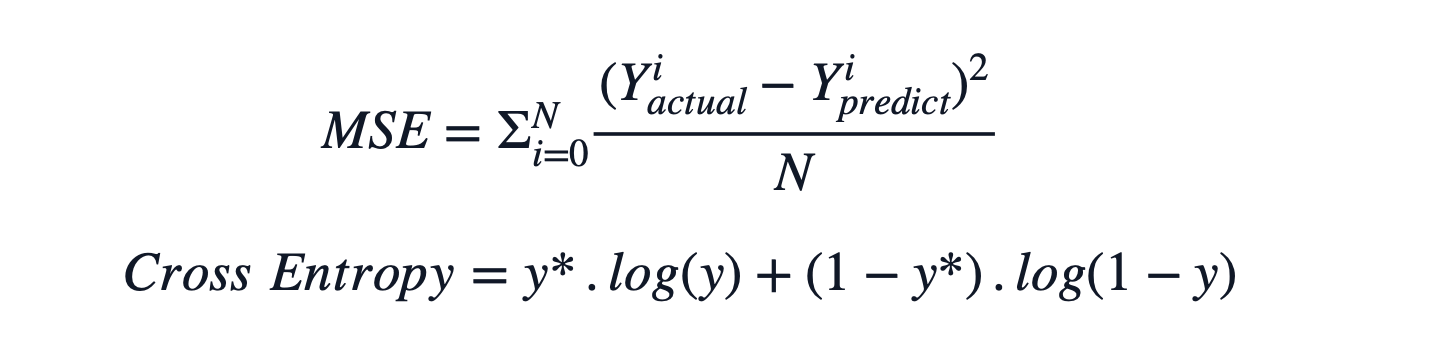#### Role of Optimization Algorithms

Likewise, in classical machine learning, Neural networks also take the help of optimization algorithms to find the optimum values for the parameters to learn. At this optimum value for parameters, the cost function becomes minimum, and machines store these parameters in the form of weight and bias matrices as learning. The most popular optimization algorithm in machine learning is the Gradient Descent algorithm, and we have explained it's working here in this blog: Gradient Descent in Machine Learning.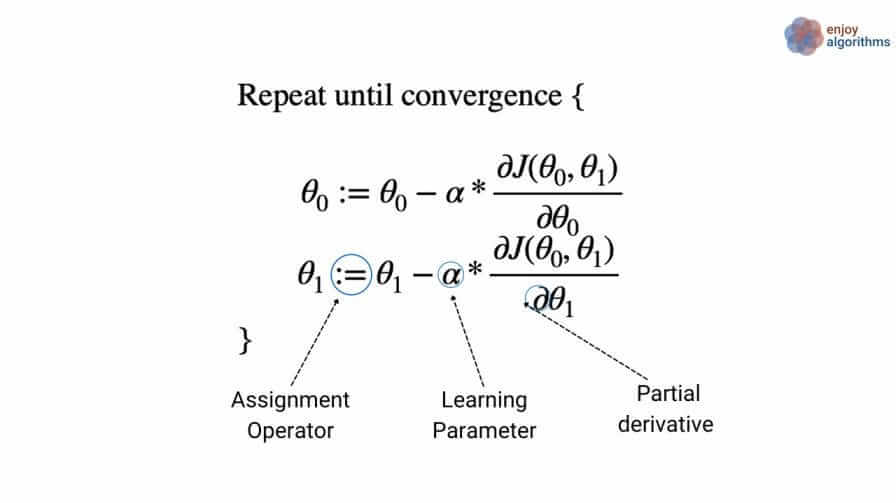In the equation above, the primary thing to notice is calculating the partial derivative for the cost function “J” w.r.t. the involved parameters θs. If we calculate this term, our backpropagation will be complete.

One crucial thing to recall from the partial derivative of the cost function is that the calculations involved in classical machine learning are more straightforward than the Artificial Neural Networks. The reason for that is the presence of non-linear activation functions in the hidden and the output layer. Hence, gradient calculation becomes lengthy.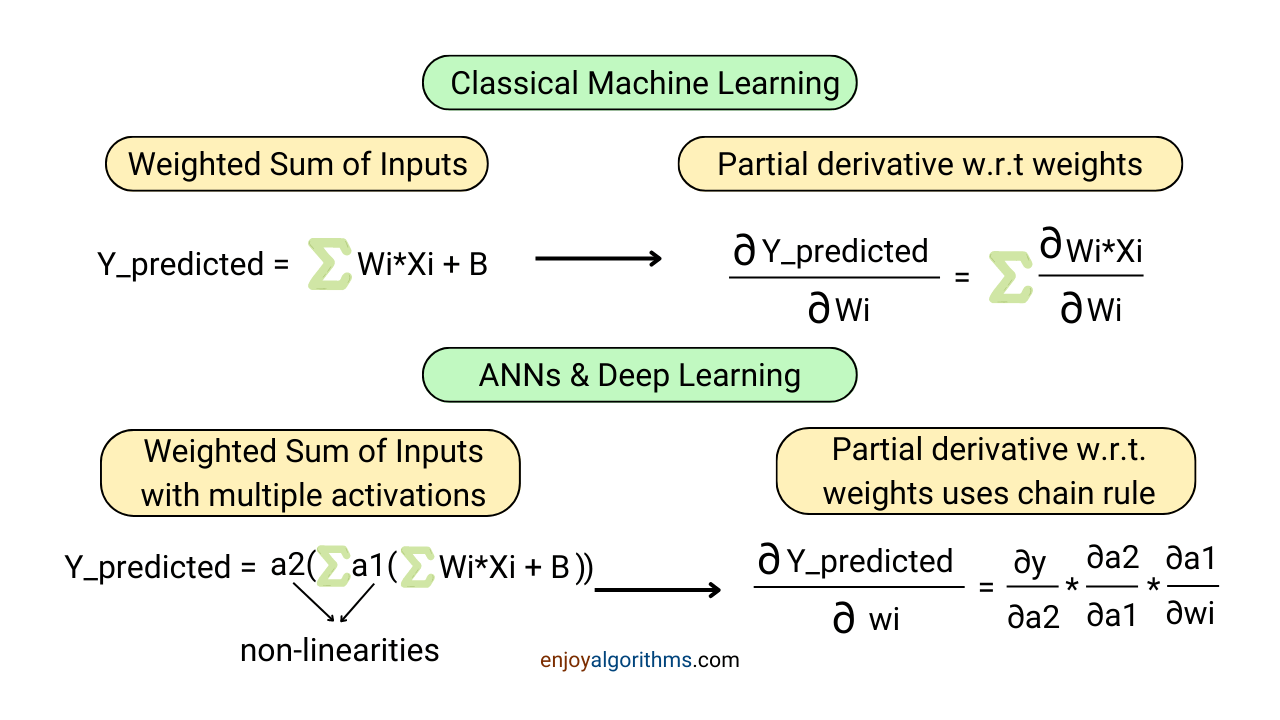#### Chain Rule and the Partial Derivatives

In ANNs, the final output we predict and use to calculate the cost becomes a complicated composite function. When we differentiate a cost function, predicted values also become part of that calculation, and the only way to differentiate a composite function is to use the chain rule. The details of this topic are covered in our chain rule for neural networks and deep learning blog. In this blog, we have covered an end-to-end use of chain rule on a network with 1 hidden layer containing 1 node.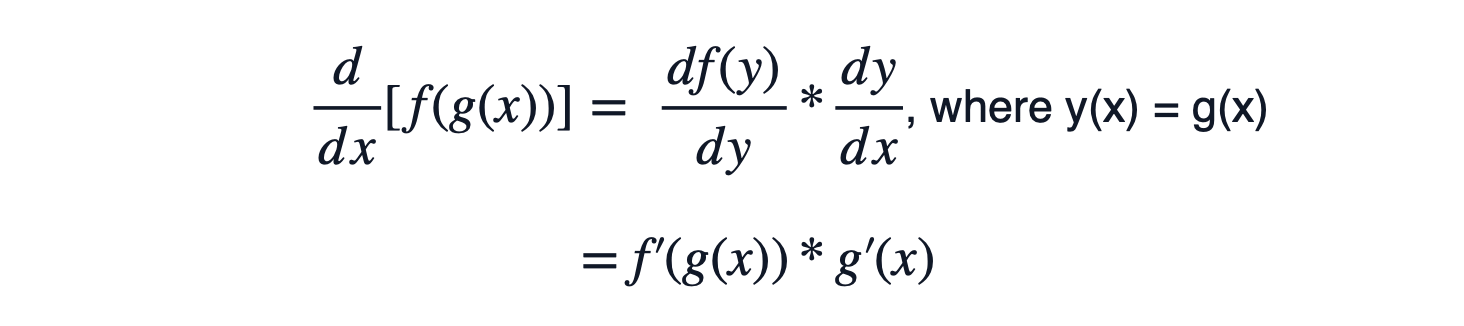For a complete understanding of backpropagation, let’s finalize things we decided till this point.

#### Defining a Neural Network Architecture

• Input data: Containing 2 features (x1, x2)
• Problem statement: Binary Classification problem (e.g., Yes, or No)
• Hidden Layer/s: There will be 1 hidden layer with 3 nodes and sigmoid activation function.
• Output Layer: As the problem is a binary classification problem, the output layer can use 1 node with the sigmoid activation function.
• Objective function/ Cost function: Let’s choose the Binary Cross Entropy as the objective function, a popular and standard function for binary classification problems.
• Optimization Algorithm: Let’s select the basicGradient Descent algorithm to see the effect of gradient calculation and its use.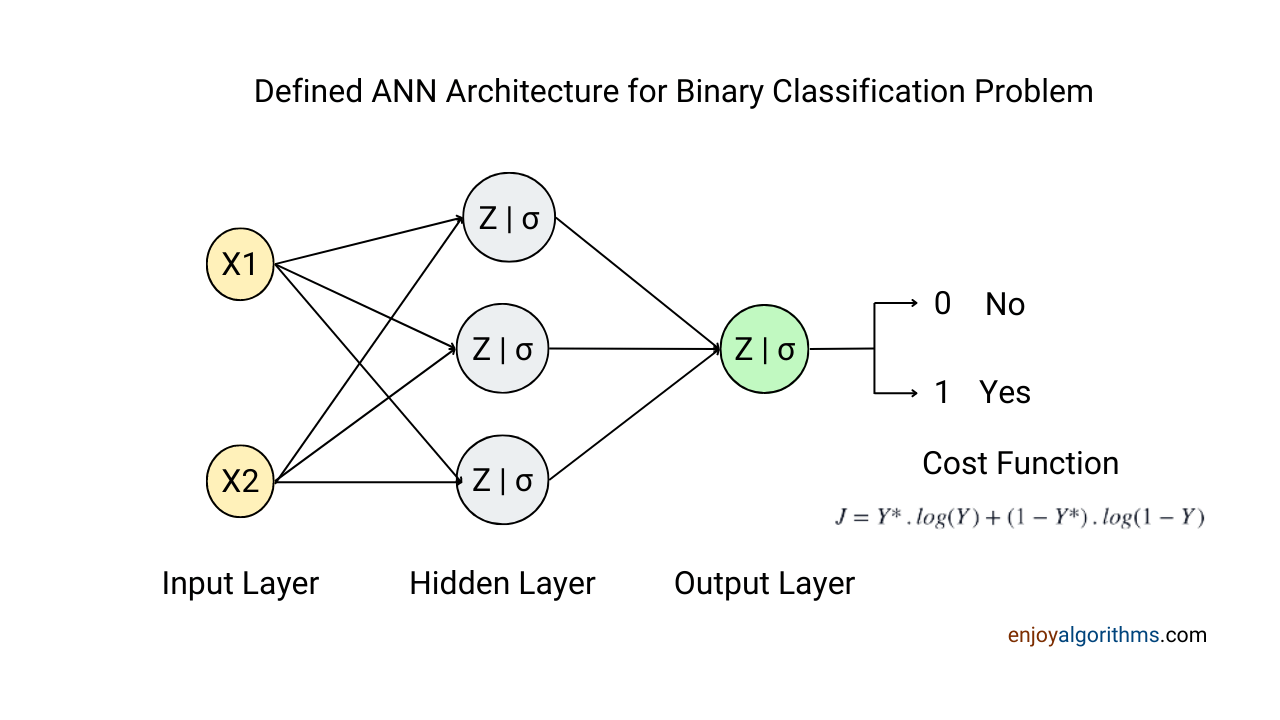### Backpropagation in neural network with an example of above architecture

A data sample passes through multiple rounds of forward and backward passes, and in each pass, optimization algorithms tune the learnable parameters to make the cost function minimum. Backpropagation is the step in ANN which is responsible for calculating the gradient of the cost function with respect to the learnable parameters. Later these gradients are utilized by the optimization algorithms to update parameters.

In all the fields of Machine Learning, there are mainly two types of parameters:

• Learnable Parameters: Weight and Bias matrices that machines learn. These parameters participate in the gradient calculation in backpropagation and optimization algorithms.
• Non-Learnable Parameters: Hyperparameters like the number of layers and the learning rate. In ML, these values are hard-coded inside the code and not learned through data samples.

#### The passing of Input samples in the topologic order

Let’s first transform the data sample from input to output step-wise. One can correlate the structure of a neural network with a tree structure where each input sample passes through each node in the topological order.

Neural networks are supervised learning algorithms, and while defining them in our programs, we need to define each node to form a directed acyclic graph. A directed graph is where every connection between the nodes (vertices) is directed from one node to another. If following the directed direction does not form any cycle (revisiting the same node), it becomes a directed acyclic graph.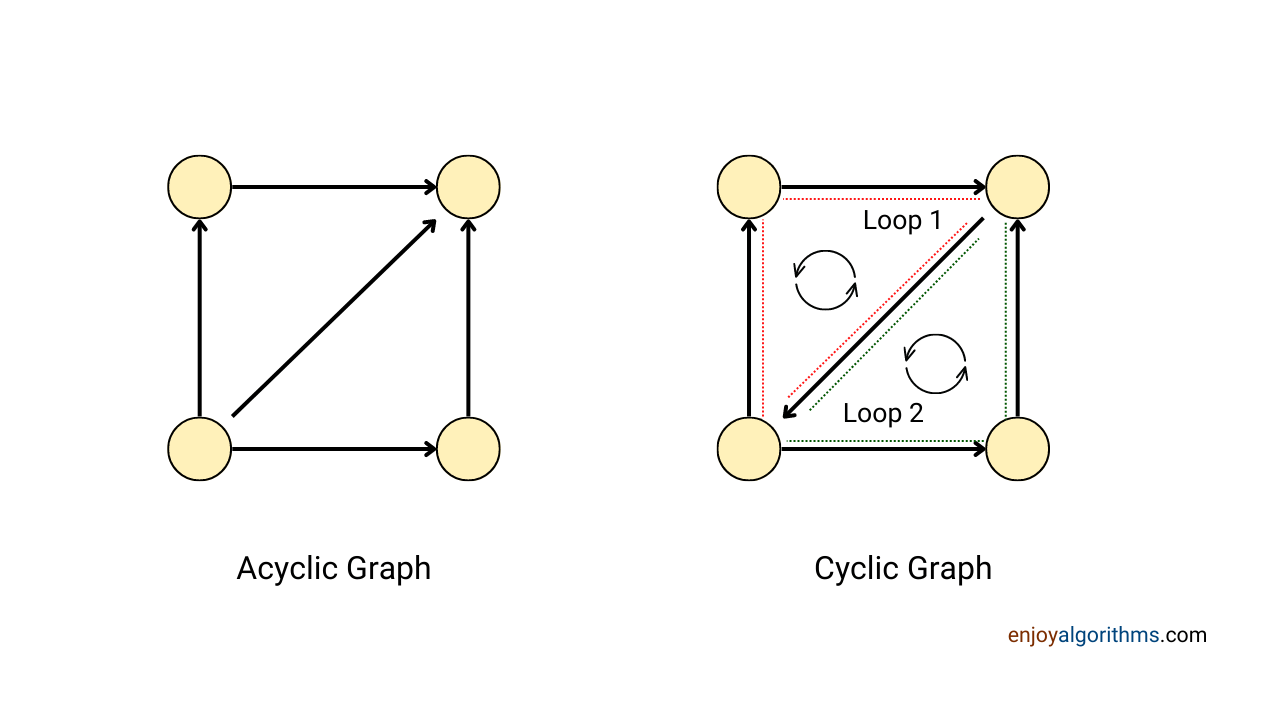In the image below, we have shown various stages involved in forward propagation. The two major transformations to the input data occur in the Hidden layer (Linear + Sigmoid) and the Ouptut layer (Linear + Sigmoid) stages. The mathematical functions for these transformations are written in the image. One can visit the blog on forward propagation for more mathematical details.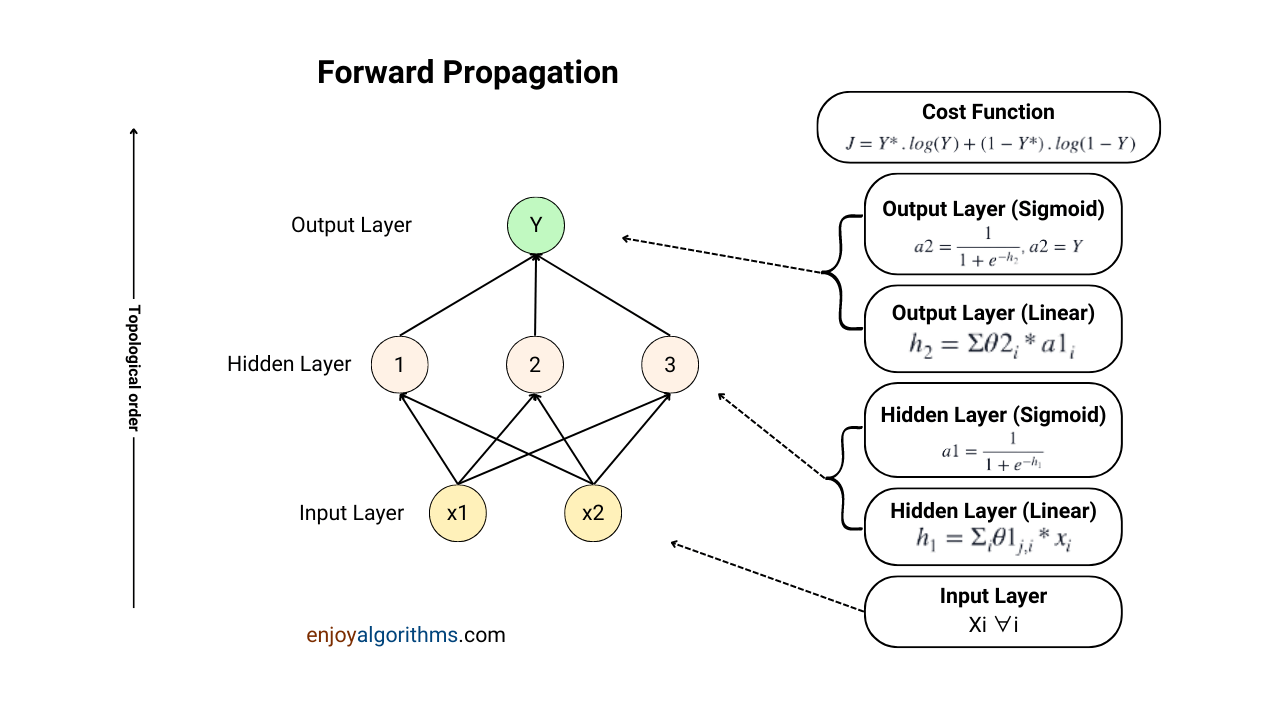The pseudocode for this approach would be:

``````Write an algorithm for evaluating function Y = f(x).
This algorithm will include the definition of each node to form an acyclic graph.

Randomly Initialize weights for each connection between two nodes
and biases for each node in the tree.

Visit all nodes of the graph in topological order

1. Compute the transformation on input after passing through that node
2. Store the values of weight and bias matrices for that node``````

Estimation of the cost function is not a part of forward propagation. So in backpropagation, the first step would be calculating the cost function. The selected loss function in terms of Y* ( Yactual) and Y (Ypredicted) will be

``J = Y* . log(Y) + (1-Y*) . log (1-Y)``

This loss will play a significant role in updating all the learnable parameters involved in forward propagation. We will calculate the gradients of the cost function w.r.t. the parameters, but this time in reverse topological order (Output to Input).

If we carefully notice the calculations of forward propagation, there are only two weight types (θ1(i,j) & θ2(i,j)), where θ1(1, 1) represents the weight of the connection between x1, and first node (out of 3 nodes) in the hidden layer. In this specific example, we have kept the values for Biases as 0 for simplification. So, our cost function needs to be only differentiated with respect to variants of θ1 and θ2. But J is represented in terms of Y and not θs. That’s where we will use the chain rule of calculus.

Calculations of gradients at various stages are shown in the image below and one can find the difference in calculations involved in forward and backward propagation. Let’s discuss each of these steps in detail.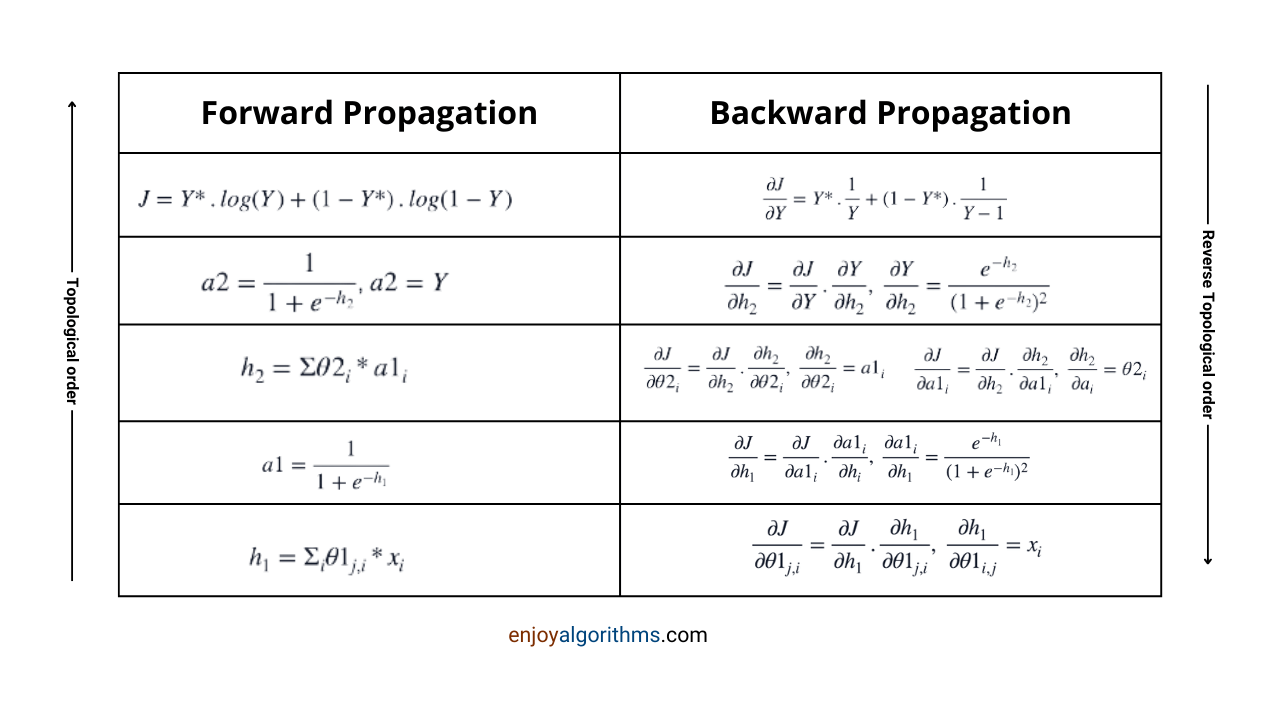Step 1: Calculating the partial derivative of the cost function w.r.t. Y. This gradient will be utilized while calculating the gradients with respect to θ1 and θ2.

``````∂J/∂Y = Y* . ∂log(Y)/∂Y + (1-Y*) . ∂log (1-Y)/∂Y

∂J/∂Y = Y*. 1/Y + (1-Y*) . 1/(Y-1)``````

Step 2: Now, Y can be written in terms of h2 as,

``a2 = Y = 1/(1+exp(-h2))``

If we differentiate this sigmoid activation function w.r.t. to h2, it will look like this:

``∂Y/∂h2 = exp(-h2)/(1+exp(-h2))^2``

Now, h2 is a function of θ2, and hence we need to find the derivative of h2 w.r.t. θ2i, which will result in a1i.

``````h2 = Σθ2i.a1i = θ21.a11 + θ22.a12 + θ23.a13

∂h2/∂θ2i = a1i``````

This will solve the purpose of differentiating the cost function J w.r.t. θ2 via the chain rule like this:

``````L.H.S. = R. H. S.

∂J/∂θ2i = ∂J/∂Y * ∂Y/∂h2 * ∂h2/∂θ2i``````

All the values in the RHS are known, so we can easily calculate the LHS part.

Step 3: Half part is done for gradient calculations. The next thing left is calculating the partial derivative of cost function J, w.r.t. θ1i. For this, we will have to go ahead with more chains, making things interesting. If we have to increase the chain beyond ∂J/∂θ2i, the next calculation would involve the differentiation of θ2i w.r.t. variable/s present in the nodes of the hidden layer.

But the exciting part is θ2i is not dependent on the variables involved in the hidden layer nodes. So the derivative will become zero, right? But if the derivative becomes zero, the chain rule will break. That’s interesting!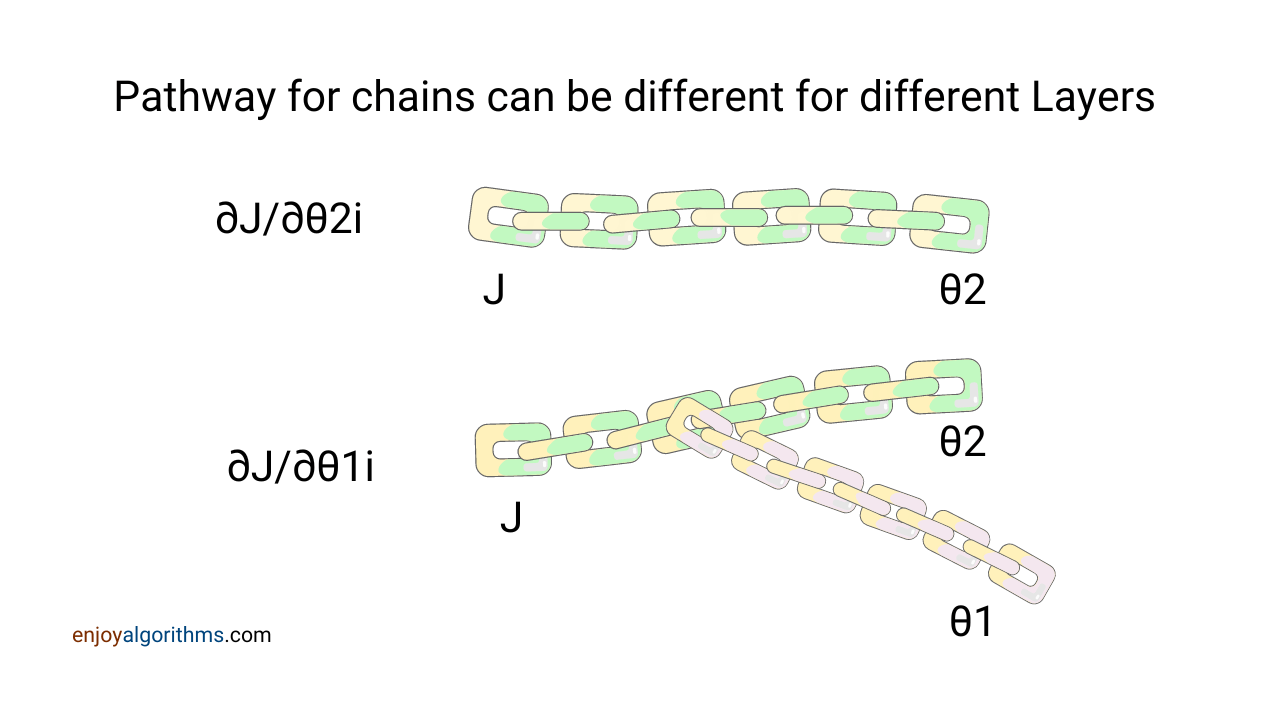To get out of this puzzle, we will form a new chain after ∂Y/∂h2. ‘h2’ is not only varying with θ2 but also with a1i, and we can take the route of a1i to reach θ1 variable and calculate the partial derivative. So, let’s estimate ∂h2/∂a1i:

``````h2 = Σθ2i.a1i = θ21.a11 + θ22.a12 + θ23.a13

∂h2/∂a1i = θ2i``````

Step 4: Now, ‘a1i’ can be represented as ‘h1i’, and we can increase the chain till h1i.

``````a1i = 1/(1+exp(-h2i))

∂a1i/∂h1 = exp(-h1)/(1+exp(-h1))^2``````

After this, as h1 can be represented using θ1(j, i), let’s quickly find the partial derivative of h1 w.r.t. the θ1, and our job will be done.

``````h1 = Σθ1(j,i).xi

∂h1/θ1(j,i) = xi

L.H.S. = R.H.S.

∂J/∂θ1i = ∂J/∂Y * ∂Y/∂h2 * ∂h2/∂a1i * ∂a1i/∂h1 * ∂h1/θ1(j,i)``````

As we know all the terms in the RHS, we can easily calculate the LHS part.

#### Psuedo-code for Backpropagation

``````Visit each node of neural network in reverse topological order

Get the representation of variable J in terms of output Y and calculate ∂J/∂Y

For all learnable paramaters:

Represent Y in terms of previous variable and extend the chain rule until
the learnable paramters``````

This completes the backward propagation for our defined neural network architecture. But before wrapping this session on backpropagation, let’s quickly see how these gradients will be used later.

#### How will backpropagation help neural networks or deep learning algorithms?

Once these gradients are calculated, the job of the optimization algorithm will start. We have selected the basic gradient descent as our optimization algorithm; let’s see how it will use these gradients. The Pseudo code for the gradient descent algorithm is:

``````repeat until convergence {

for all i:
θ1i = θ1 - α * ∂J/∂θ1i

for all i:
θ2i = θ2i - α * ∂J/∂θ2i

}``````

In the update part of the equation, θ1-α * ∂J/∂θ1i, gradient helps optimization algorithms to decide whether to increase or decrease parameters. If the gradient is +ve, θ will be reduced to achieve the minimum of the cost function. Similarly, if the gradient is -ve, θ will be increased to achieve a minimum of the cost function.

That’s it for this blog, and we hope you enjoyed the article.

#### Conclusion

Backpropagation or backward propagation is a step in neural networks that gets executed only at the time of training and is responsible for calculating the gradients of the cost function with respect to the learnable parameters. It is considered one of the most important and renowned topics in ANN, which we discussed in this blog. In the last, we also saw the application of the chain rule in backpropagation to compute gradients. If you are reading this, we hope you enjoyed the article.

##### Enjoy Learning! Enjoy Algorithms!

☆ 16-Week Live DSA Course
☆ 10-Week Live DSA Course